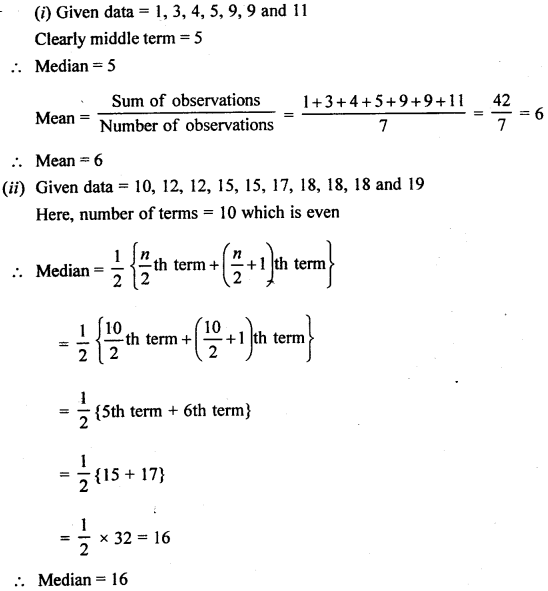# Selina Concise Mathematics Class 6 ICSE Solutions Chapter 34 Mean and Median

## Selina Concise Mathematics Class 6 ICSE Solutions Chapter 34 Mean and Median

Selina Publishers Concise Mathematics Class 6 ICSE Solutions Chapter 34 Mean and Median

### Mean and Median Exercise 34A – Selina Concise Mathematics Class 6 ICSE Solutions

Question 1.
Find the mean of :
(i) 7,10, 4 and 17
(ii) 12, 9, 6,11 and 17
(iii) 3, 1, 5, 4, 4 and 7
(iv) 7, 5, 0, 3, 0, 6, 0, 9, 1 and 4
(v) 2.1, 4.5, 5.2, 7.1 and 9.3
(vi) 5, 2.4, 6.2, 8.9, 4.1 and 3.4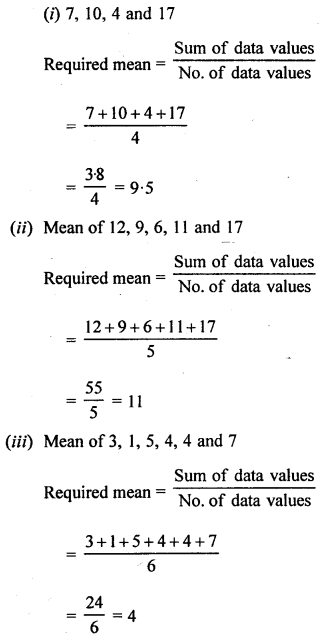Question 2.
Find the mean of :
(i) first eight natural numbers
(ii) first six even natural numbers
(iii) first five odd natural numbers
(iv) all prime numbers upto 30
(v) all prime numbers between 20 and 40.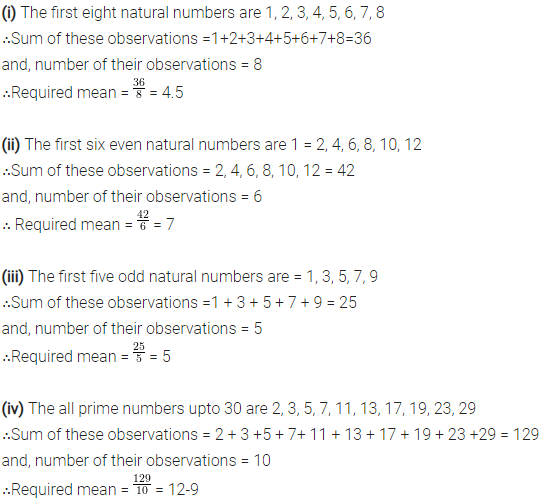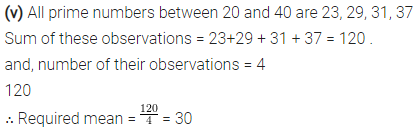Question 3.
Height (in cm) of 7 boys of a locality are 144 cm, 155 cm, 168 cm, 163 cm, 167 cm, 151 cm and 158 cm. Find their mean height.Question 4.
Find the mean of 35, 44, 31, 57, 38, 29, 26,36, 41 and 43.Question 5.
The mean of 18, 28, x, 32, 14 and 36 is 23. Find the value of x.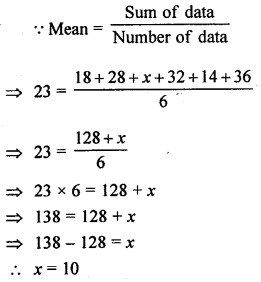Question 6.
If the mean of x, x + 2, x + 4, x + 6 and x + 8 is 13, find the value of x.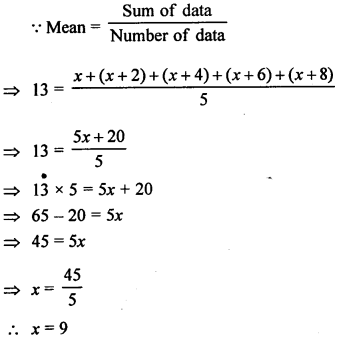### Mean and Median Exercise 34B – Selina Concise Mathematics Class 6 ICSE Solutions

Question 1.
Find the median of
(i) 21, 21, 22, 23, 23, 24, 24, 24, 24, 25 and 25
(ii) 3.2, 4.8, 5.6, 5.6, 7.3, 8.9 and 91
(iii) 17, 23, 36, 12, 18, 23, 40 and 20
(iv) 26, 33, 41, 18, 30, 22, 36, 45 and 24
(v) 80, 48, 66, 61, 75, 52, 45 and 70
Solution: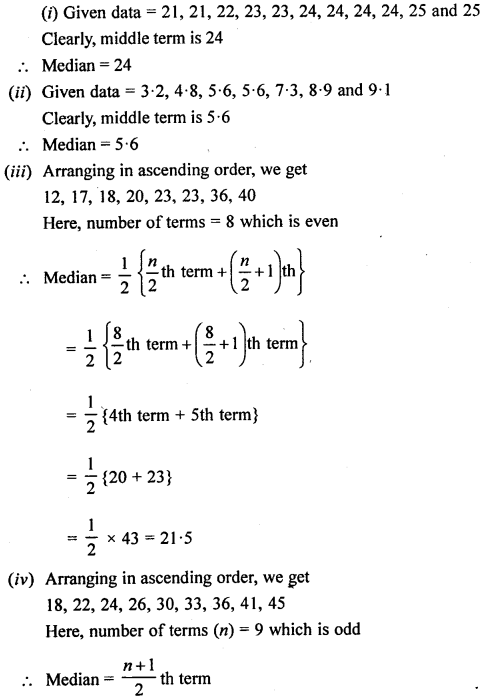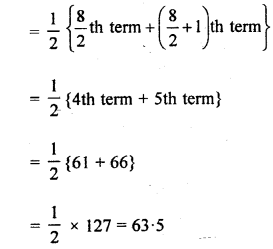Question 2.
Find the mean and the median of :
(i) 1,3,4, 5, 9, 9 and 11
(ii) 10,12, 12, 15, 15, 17, 18, 18, 18 and 19
(iii) 2, 4, 5, 8, 10,13 and 14
(iv) 5, 8, 10, 11,13, 16, 19 and 20
(v) 1.2, 1.9, 2.2, 2.6 and 2.9
(vi) 0.5, 5.6, 3.8, 4.9, 2.7 and 4.4.
Solution: